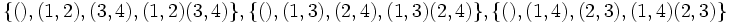# Difference between revisions of "Subgroup structure of symmetric group:S4"

The symmetric group on four letters has many sugbroups.

Note that since$S_4$ is a complete group, every automorphism is inner, so the classification of subgroups upto conjugacy is equivalent to the classification of subgroups upto automorphism. In other words, every subgroup is an automorph-conjugate subgroup.

1. The trivial subgroup. Isomorphic to trivial group.(1)
2. The two-element subgroup generated by a transposition, such as$(1,2)$. Isomorphic to cyclic group of order two.(6)
3. The two-element subgroup generated by a double transposition, such as$(1,2)(3,4)$. Isomorphic to cyclic group of order two. (3)
4. The four-element subgroup generated by two disjoint transpositions, such as$\langle (1,2) \ , \ (3,4) \rangle$. Isomorphic to Klein-four group. (3)
5. The unique four-element subgroup comprising the identity and the three double transpositions. Isomorphic to Klein-four group. (1)
6. The four-element subgroup spanned by a 4-cycle. Isomorphic to cyclic group of order four.(3)
7. The eight-element subgroup spanned by a 4-cycle and a transposition that conjugates this cycle to its inverse. Isomorphic to dihedral group of order eight. (3)
8. The three-element subgroup spanned by a three-cycle. Isomorphic to cyclic group of order three.(4)
9. The six-element subgroup comprising all permutations that fix one element. Isomorphic to symmetric group on three elements. (4)
10. The alternating group: the subgroup of all even permutations. Isomorphic to alternating group:A4.(1)
11. The whole group.(1)

## The alternating group (twelve-element characteristic subgroup) (type (10))

This is a characteristic subgroup. The alternating group inside the symmetric group on the set$\{ 1,2,3,4 \}$ is given as:$\{ (), (1,2,3), (1,3,2), (1,2,4), (1,4,2), (2,3,4), (2,4,3), (1,3,4), (1,4,3), (1,3)(2,4), (1,4)(2,3), (1,2)(3,4) \}$

### Subgroup-defining functions yielding this subgroup

• The commutator subgroup.
• The Jacobson radical: It is, in fact, the unique maximal normal subgroup.
• The subgroup generated by squares.
• The Sylow-closure for the prime$3$.

### Subgroup properties satisfied by this subgroup

The alternating group is a verbal subgroup on account of being generated by commutators, or equivalently, on account of being generated by squares (actually, the two facts are closely related, and have to do with the fact that the symmetric group is a group generated by involutions). Thus, it satisfies some subgroup properties, including:

It also satisfies some other subgroup properties, such as:

## The Klein-four group of double transpositions (type (5))

This is a characteristic subgroup. In the symmetric group on the set$\{ 1,2,3,4\}$, it is given as:$\{ (), (1,2)(3,4), (1,3)(2,4), (1,4)(2,3) \}$

### Subgroup properties satisfied by this subgroup

The given subgroup is a verbal subgroup on account of being the second member of the derived series. Thus, it satisfies some subgroup properties, including:

It also satisfies some other subgroup properties, such as:

### Subgroup properties not satisfied by this subgroup

This subgroup can be obtained as follows. Consider the Klein-four group, and use the embedding of this group inside the symmetric group on four letters given by Cayley's theorem. The image is precisely this subgroup.

## The two-element subgroup generated by a transposition (type (2))

There are six such subgroups. In the symmetric group on$\{ 1,2,3,4\}$, these subgroups are given by:$\{ (), (1,2) \}, \{ (), (2,3) \}, \{ (), (1,3) \}, \{ (), (1,4), \}, \{ (), (2,4) \} \{ (), (3,4) \}$

### Effect of operators

• Centralizer: The centralizer is a Klein-four group, of type (4).
• Normalizer: The normalizer is a dihedral group of order eight, of type (7).

## The two-element subgroup generated by a double transposition (type (3))

There are three such subgroups. In the symmetric group on$\{ 1,2,3,4\}$, these are given by:$\{ (), (1,2)(3,4) \}, \{ (1,3)(2,4) \}, \{ (1,4)(2,3) \}$.

These three subgroups are in bijection with the three subgroups of type (4) via the centralizer operation, and also with the three 2-Sylow subgroups of order eight (type (7)) via the normalizer operation.

### Effect of operators

• Normal closure: The normal closure is the Klein-four group, type (5).
• Normalizer: The normalizer is a dihedral group. Each of these subgroups has a different dihedral group as its normalizer.
• Centralizer: The centralizer is the same as the normal closure.

## The non-normal Klein-four groups (type (4))

There are three such groups. These three groups are in bijection with the three subgroups of type (3) discussed above via the centralizer operation, and also with the three 2-Sylow subgroups, via the normalizer operation.$\{ (), (1,2), (3,4), (1,2)(3,4) \}, \{ (), (1,3), (2,4), (1,3)(2,4) \}, \{ (), (1,4), (2,3), (1,4)(2,3) \}$